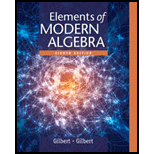Elements Of Modern Algebra
8th Edition
ISBN: 9781285463230
Author: Gilbert, Linda, Jimmie
Publisher: Cengage Learning,Elements Of Modern Algebra
Fundamentals. 6TFE

# Whether the statement, “Let R be a relation on nonempty set A that is symmetric and transitive. Since R is symmetric x R y implies that y R x . Since R is transitive x R y and y R x implies that x R x . Hence R is reflexive and thus an equivalence relation on set A ” is true or false.

QuestionChapter 1.7, Problem 6TFE
To determine

## Whether the statement, “Let R be a relation on nonempty set A that is symmetric and transitive. Since R is symmetric xRy implies that yRx. Since R is transitive xRy and yRx implies that xRx. Hence R is reflexive and thus an equivalence relation on set A ” is true or false.

### Want to see the full answer?

Check out a sample textbook solutionElements Of Modern Algebra
8th Edition
ISBN: 9781285463230
Author: Gilbert, Linda, Jimmie
Publisher: Cengage Learning,Elements Of Modern Algebra
Fundamentals. 6TFEMore videos on
RelationsWant to see this answer and more?
Experts are waiting 24/7 to provide step-by-step solutions in as fast as 30 minutes!*
*Response times may vary by subject and question complexity. Median response time is 34 minutes for paid subscribers and may be longer for promotional offers.
Knowledge BoosterNeed a deep-dive on the concept behind this application? Watch these videos for an in-depth look
What is a Relation? | Don't Memorise
Don't Memorise
RELATIONS-DOMAIN, RANGE AND CO-DOMAIN (RELATIONS AND FUNCTIONS CBSE/ ISC MATHS)
Neha Agrawal Mathematically Inclined
Recommended textbooks for you
•Elements Of Modern Algebra
Algebra
ISBN:9781285463230
Author:Gilbert, Linda, Jimmie
Publisher:Cengage Learning,
•Elements Of Modern Algebra
Algebra
ISBN:9781285463230
Author:Gilbert, Linda, Jimmie
Publisher:Cengage Learning,